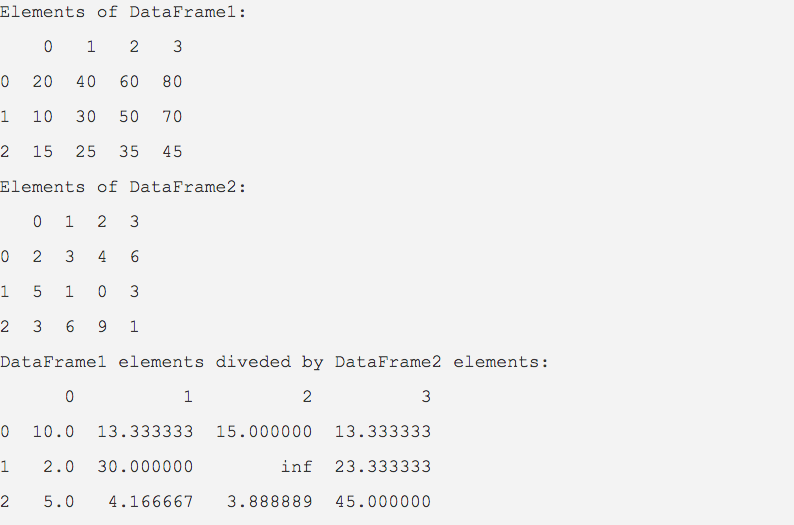# Dataframe Division Operations

## Overview:

• div() method divides element-wise division of one pandas DataFrame by another.
• DataFrame elements can be divided by a pandas series or by a Python sequence as well.
• Calling div() on a DataFrame instance is equivalent to invoking the division operator (/).
• The div() method provides the fill_value parameter which is used for replacing the np.nan and None values present in the DataFrame or in the resultant value with any other value.

## Example:

 import pandas as pd   # Data creation part data1 = [(20, 40, 60, 80),          (10, 30, 50, 70),          (15, 25, 35, 45)];   data2 = [(2, 3, 4, 6),          (5, 1, 0, 3),          (3, 6, 9, 1)];   # Creation of pandas DataFrames dataFrame1 = pd.DataFrame(data=data1); dataFrame2 = pd.DataFrame(data=data2);   # Divide the DataFrame1 elements by the elements of DataFrame2 divisionResults = dataFrame1.div(dataFrame2);   print("Elements of DataFrame1:") print(dataFrame1);   print("Elements of DataFrame2:") print(dataFrame2);   print("DataFrame1 elements divided by DataFrame2 elements:") print(divisionResults);

## Output: